# Pythagorean theorem - math problems

#### Number of problems found: 792

• Find the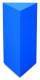Find the weight of a wooden regular triangular prism with a height equal to the perimeter of the base and a figure inscribed in a circle with a radius of 6, M cm, where M is the month of your birth. The density of oak is 680 kg/m ^ 3.
• Isosceles triangle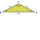Calculate the height of the isosceles triangle ABC with the base AB, AB = c = 10 cm and the arms a = b = 13 cm long.
• Trapezoid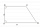The rectangular trapezoid ABCD with right angle at the vertex A has sides a, b, c, d. Calculate the circumference and the area of the trapezoid if given: a = 25cm, c = 10cm, d = 8cm
• The block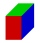The block has the dimensions of 5 cm, 10 cm, and 15 cm. Calculate the size of the wall diagonals of this block.
• Angle ASB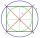On a circle with a radius of 10 cm and with a center S, the points A, B, C are given so that the central angle ASB is 60 degrees and the central angle ASC is 90 degrees. Find the length of the circle arc and the amount of AB and AC offsets.
• A paint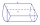A paint tin is a cylinder 12cm and height 22 cm. Leonardo, the painter, drops his stirring stick into the tin and it disappears. Work out the maximum length of the stick.
• Circle inscribed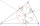There is a triangle ABC and a circle inscribed in this triangle with radius 15. The point T is the point of contact of the inscribed circle with the side BC. What is the area of the triangle ABC if | BT | = 25 a | TC | = 26?
• Touch circle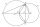Point A has a distance IA, kl = 10 cm from a circle k with radius r = 4 cm and center S. Calculate: a) the distance of point A from the point of contact T if the tangent to the circle is drawn from point A b) the distance of the contact point T from the l
• Diagonals of rhombus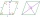Find a length of the diagonal AC of the rhombus ABCD if its perimeter P = 112 dm and the second diagonal BD has a length of 36 dm.
• One leg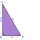One leg of a right triangle is 1 foot longer than the other leg. The hypotenuse is 5 feet. Find the lengths of the three sides of the triangle.
• The coordinates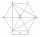The coordinates (5, 2) and (-6, 2) are vertices of a hexagon. Explain how to find the length of the segment formed by these endpoints. How long is the segment?
• Michael 2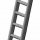Michael has a 35 foot ladder leaning against the side of his house. If the bottom of the ladder is 21 feet away from his house, how many feet above the ground does the ladder touch the house?
• Luiza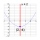Luiza delivers newspapers in her neighborhood. If you plot the points (-1, 1), (4, 1), (4, -2) and (-1, -2), you will create a representation of the route she takes, in miles. How many miles does her route cover?
• Distance two imaginary numbs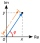Find the distance between two complex number: z1=(-8+i) and z2=(-1+i).
• Construct 8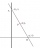Construct an analytical geometry problem where it is asked to find the vertices of a triangle ABC: the vertices of this triangle must be the points A (1,7) B (-5,1) C (5, -11). the said problem should be used the concepts of: distance from a point to a li
• Wheel gearA drive wheel of radius 2 is connected to a drive wheel of radius 1 by a pulley of length 17. What is the distance between the wheel axles?
• Centre of the hypotenuseFor the interior angles of the triangle ABC, alpha beta and gamma are in a ratio of 1: 2: 3. The longest side of the AB triangle is 30 cm long. Calculate the perimeter of the triangle CBS if S is the center of the side AB.
• MIT 1869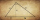You know the length of hypotenuse parts 9 and 16, at which the hypotenuse of a right triangle is divided by a perpendicular running from its opposite vertex. The task is to find the lengths of the sides of the triangle and the length of the line x. This a
• The gable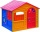The gable of the house has the shape of an isosceles triangle, which has a base length of 14 meters, and arms with a length of 8 meters. What is the tall gable of the house?
• Length of the chord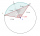Calculate the length of the chord in a circle with a radius of 25 cm with a central angle of 26°.

Do you have an exciting math question or word problem that you can't solve? Ask a question or post a math problem, and we can try to solve it.

We will send a solution to your e-mail address. Solved examples are also published here. Please enter the e-mail correctly and check whether you don't have a full mailbox.

Pythagorean theorem is the base for the right triangle calculator.